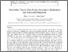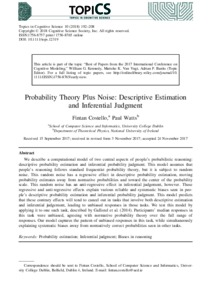# Probability Theory Plus Noise: Descriptive Estimation and Inferential Judgment

Costello, Fintan and Watts, Paul (2018) Probability Theory Plus Noise: Descriptive Estimation and Inferential Judgment. Topics in Cognitive Science, 10. pp. 192-208. ISSN 1756-8757Previewmore...Add this article to your Mendeley library

## Abstract

We describe a computational model of two central aspects of people’s probabilistic reasoning: descriptive probability estimation and inferential probability judgment. This model assumes that people’s reasoning follows standard frequentist probability theory, but it is subject to random noise. This random noise has a regressive effect in descriptive probability estimation, moving probability estimates away from normative probabilities and toward the center of the probability scale. This random noise has an anti-regressive effect in inferential judgement, however. These regressive and anti-regressive effects explain various reliable and systematic biases seen in people’s descriptive probability estimation and inferential probability judgment. This model predicts that these contrary effects will tend to cancel out in tasks that involve both descriptive estimation and inferential judgement, leading to unbiased responses in those tasks. We test this model by applying it to one such task, described by Gallistel et al. (2014). Participants’ median responses in this task were unbiased, agreeing with normative probability theory over the full range of responses. Our model captures the pattern of unbiased responses in this task, while simultaneously explaining systematic biases away from normatively correct probabilities seen in other tasks.

Item Type: Article Probability estimation; Inferential judgment; Biases in reasoning; Faculty of Science and Engineering > Theoretical Physics 13095 https://doi.org/10.1111/tops.12319 Paul Watts 23 Jun 2020 15:24 Topics in Cognitive Science Wiley Online Library YesItem control page